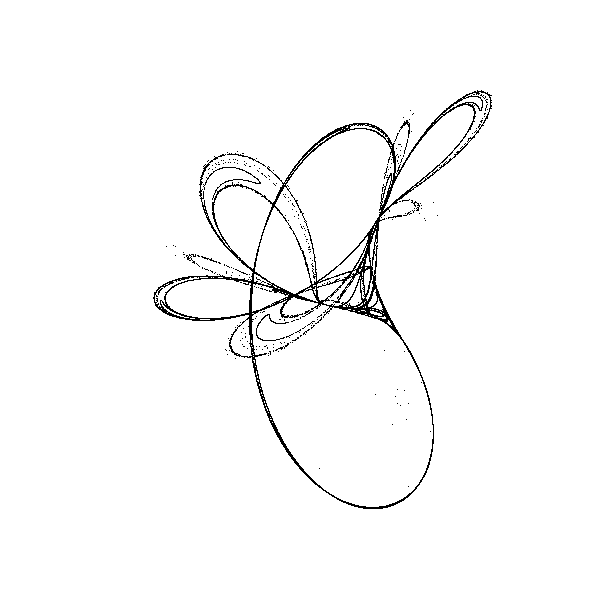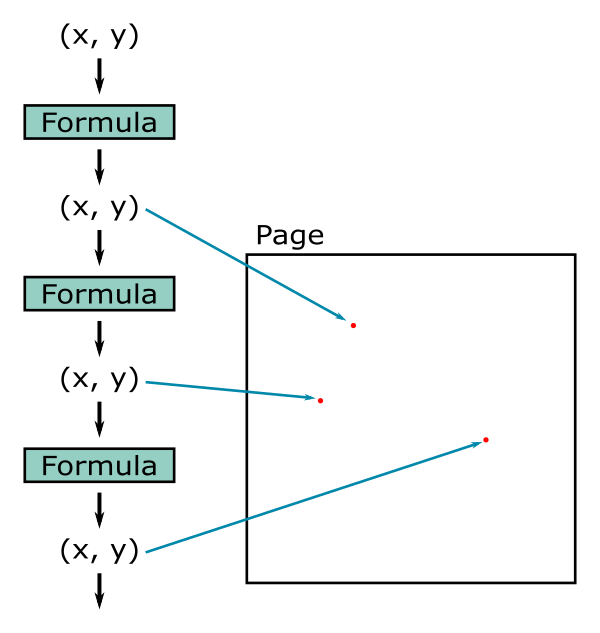# Black and white Tinkerbell fractal in generativepy

Martin McBride
2021-12-14

The Tinkerbell fractal is based on an iterated function. Here we will look at an implementation using generativepy.

Here is a simple black and white version of the fractal (the coloured version in the next article is a lot nicer):## Tinkerbell algorithm

The Tinkerbell fractal is based on two simple equations that take a value (x, y) and calculate a new value (xnext, ynext). The formulas are:

xnext = x*x - y*y + A*x + B*y
ynext = 2*x*y + C*x + D*y


Suitable parameters are:

A = 0.9
B = -0.6013
C = 2.0
D = 0.5

x = 0.01  # Initial value
y = 0.01  # Initial value


We start with the initial value (0.1, 0.1). We place a dot on the page at this position.

Next, we feed those values into the equations above, which creates news x and y values (0.002987, 0.0252). We place another dot at that position.

We then feed those new values back into the same equations, which creates news x and y values (-0.013090577831 0.0187245448). We place another dot at that position.

This process is illustrated here:We repeat this procedure many more times. The next few values of x and y are:

-0.023219834186157737 -0.017309113484108886
-0.01025032553952039 -0.05429039562434912
0.020577144019970572 -0.046532860433577475


These positions all seem quite random. However, if you plot thousands of points, a pattern emerges as we saw above. Even though each new pixel seems to be in a random position all the pixels end up in a particular part of the image. It is as if they all seem to land on a particular, complicated curved shape.

It is stranger than that. If you look at some of the loops in the curve, you will see that there are several separate lines that each follow a similar path. But if we were to zoom in further, we would find that each of those lines is made up of several different lines, very close together. And if we zoomed in even further, each of those lines would be made up of several different lines, even closer together. There is an infinite level of detail within the image, you could zoom in forever and never get to the end.

We call this shape a strange attractor. Attractor because it appears to attract all the points to it, and strange because such a complex shape has been created from such a simple formula.

You can vary the values of the parameters to create different variants of the fractal. It will usually create a fractal of some type, but not all values will create pleasing images.

## Drawing the fractal in generativepy

generativepy gives you several drawing options. For fractals like this one that involve setting individual pixels, the best way is to use the nparray module. This represents an image as a NumPy array, which allows you to access pixels very efficiently, as well as giving you lots of other useful processing options. The NumPy array is ultimately saved as a PNG image.

In this case, we will use a greyscale NumPy array, which is an int array of dimensions height by width by 1. Each element is an integer value between 0 (black) and 255 (white).

Remember that NumPy stores elements in rows then columns, so the first value is the y coordinate (the row) and the second value is the x coordinate (the column). This also means that this size is expressed as height then width.

## The code

Here is the full code of the fractal:

from generativepy.bitmap import Scaler
from generativepy.nparray import make_nparray

MAX_COUNT = 100000
A = 0.9
B = -0.6013
C = 2.0
D = 0.5

def paint(image, pixel_width, pixel_height, frame_no, frame_count):
scaler = Scaler(pixel_width, pixel_height, width=3, startx=-2, starty=-2)

x = 0.01
y = 0.01
for i in range(MAX_COUNT):
x, y = x*x - y*y + A*x + B*y, 2*x*y + C*x + D*y
px, py = scaler.user_to_device(x, y)
image[py, px] = 0

make_nparray('tinkerbell-bw.png', paint, 600, 600, channels=1)


This code is available on github in blog/fractals/tinkerbell-bw.py.

We are using make_nparray to create the image, with a size of 600 by 600, and 1 channel (ie greyscale). This function calls our paint function, passing in a NumPy array that we can fill without pixel values. The array is prefilled with the value 255 (white). If we set any array value to 0, it will cause that pixel in the image to be black.

The Tinkerbell formula creates values that have x and y values between -2 and +1. To create a suitable image, we need to map these x and y values onto equivalent pixel positions in the range 0 to 599 (since our image is 600 pixels square). We use a Scaler to convert x and y values into pixel positions, using the user_to_device function. See the Scaler specification.

In the main loop of the paint function, we loop 100,000 times, calculating new x and y values and setting the corresponding pixel to black. make_nparray then writes this array out as an image file tinkerbell-bw.png, which you can see at the start of this article.